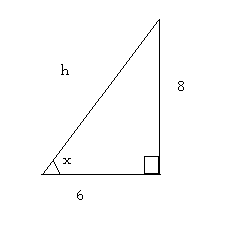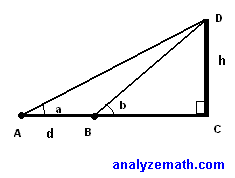# Solve Right Triangle Problems

Right triangles problems are solved and detailed explanations are included.

Example - Problem 1: Find sin(x) and cos(x) in the right triangle shown below.Solution to Problem 1:

1. First use the
Pythagorean theorem to find the hypotenuse h of the right triangle.

h = √(62 + 82)
= √(36 + 64)
= 10
2. In a right triangle, using trigonometric ratio for sin(x) we write.
sin(x) = 8 / 10 = 0.8
3. and using trigonometric ratio for cos(x) we write
cos(x) = 6 / 10 = 0.6

Example - Problem 2: Two lines tangent to a circle at points M and N have a point of intersection A. The size of angle MAN is equal to x degrees and the length of the radius of the circle is equal to r. Find the distance from point A to the center of the circle in terms of x and r.Solution to Problem 2:

1. A line through the center C of the circle and a point of tangency to the circle is perpendicular to the tangent line, hence the right angles at M and N in the figure below.2. Triangles MAC and NAC are congruent right triangles. tan(x/2) is given by.
tan(x/2) = r / d(NA) , where d(NA) = distance between points A and N.
3. solve for d(NA)
d(NA) = r / tan(x/2)
4. Use the Pythagorean theorem in the right triangle NAC to find the distance d(AC)
d(AC) = √[ r2 + d(NA)2]
5. Substitute d(NA) by r / tan(x/2) to obtain d(AC).
d(AC) = √[ r2 + (r / tan(x/2))2]
6. Factor r2
d(AC) = √[ r2(1 + 1 / tan(x/2)2]
7. Simplify
d(AC) = r √[ 1 + 1 / tan(x/2)2]

Example - Problem 3: Two right triangles have side a in common. x is the size of angle BAC. Find tan(x).Solution to Problem 3:

1. We first use the right triangle on the right to find a
tan(41�) = a / 15
2. a is given by.
a = 15 * tan(41�)
3. We now use the right triangle on the left to find tan(x).
tan(x) = a / 10
4. Substitute a by 15 * tan(41�) in the above.
tan(x) = 15 * tan(41�) / 10

5. Which gives
tan(x) = 1.5 * tan(41�)

Example - Problem 4: From point A, an observer notes that the angle of elevation of the top of a tower (C,D) is a (degrees) and from point B the angle of elevation is b (degrees). Points A, B and C (the bottom of the tower) are collinear. The distance between A and B is d. Find the height h of the tower in terms of d and angles a and b.Solution to Problem 4:

1. Let x be the distance between points B and C, hence in the right triangle ACD we have
tan(a) = h / (d + x)
2. and in the right triangle BCD we have
tan(b) = h / x
3. Solve the above for x
x = h / tan(b)
4. Solve tan(a) = h / (d + x) for h
h = (d + x) tan(a)
5. Substitute x in above by h / tan(b)
h = (d + h / tan(b)) tan(a)
6. Solve the above for h to obtain.
h = d tan(a) tan(b) / [ tan(b) - tan(a)]